GFG App
Open AppBrowser
Continue

Area of a circle is the space occupied by the circle in a two-dimensional plane. Area of any figure is the space inside its boundaries. Area of a circle can easily be determined using a formula, A = πr2, where r is the radius of the circle. Area of a circle is measured in square units, like m2, cm2, etc.

## What is Area of a Circle?

Area of a circle is defined as the space covered by the boundary of the circle. It is measured in square units. Area of the circle is measured using the radius of the circle. It is equal to π times the radius squared.

Area of the circle is calculated using the formula,

Area of Circle = πr2

Area of Circle = πd2 / 4

where,
d is diameter
π = 22/7 or 3.14

Area of the circle formula is useful for measuring areas of circular fields or plots. It is also useful to measure the area covered by circular furniture and other circular objects.

## What is a Circle?

Circle is a collection of points that are at a fixed distance from a particular point. Every line passing through the circle forms the line of reflection symmetry. In addition to this, it has rotational symmetry around the center for every angle. Some examples of circles are wheels, pizzas, circular ground, etc. The distance from the center to the circle is known as the radius.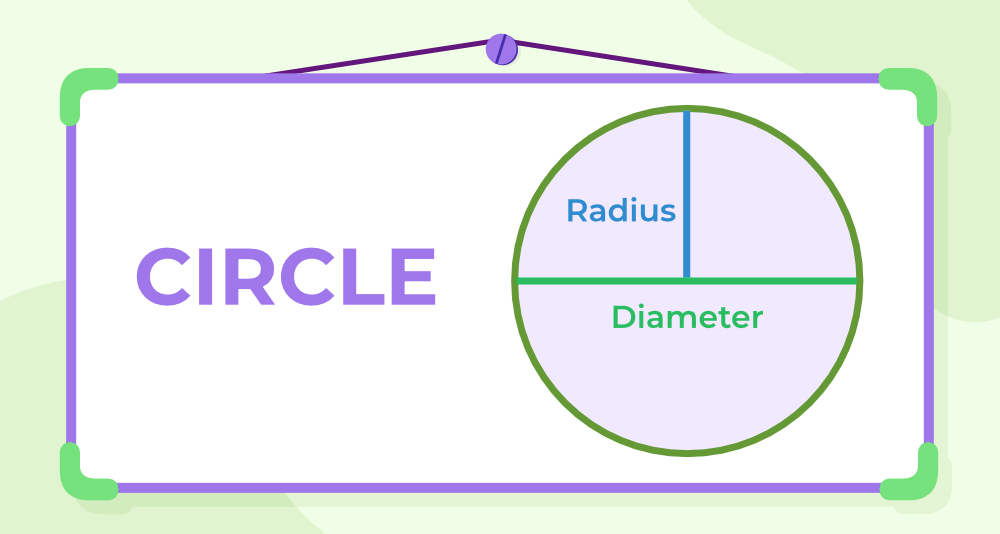## Parts of a Circle

Circle is a closed curve in which all the points are equidistant from one fixed point i.e. centre. Examples of circles as seen in everyday life are clocks, wheels, pizzas, etc. Various terms related to the circle are discussed below:

Radius: The distance of a point from the boundary of the circle to its centre is termed its radius. Radius is represented by the letter ‘r‘ or ‘R‘. The area and circumference of a circle are directly dependent on its area.

Diameter: Longest chord of a circle that passes through its centre is termed its diameter. It is always twice its radius.

Diameter formula: The formula for the diameter of a circle is Diameter = 2 × Radius

d = 2×r or D = 2×R

also, conversely, the radius can be calculated as:

r = d/2 or R = D/2

Circumference: The circumference of the circle is the total length of its boundary i.e. perimeter of a circle is termed its circumference. The Circumference of a circle is given by the formula C = 2πr.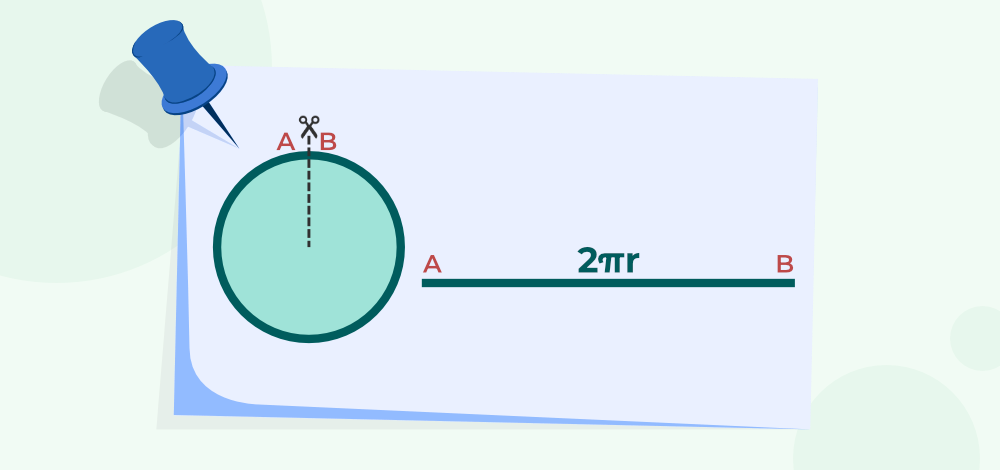## Area of Circle Formulas

The formula for finding an area of a circle is directly proportional to the square of its radius. It can also be found out if the diameter or circumference of a circle is given. Area of a circle is calculated by multiplying the square of the radius by π. Formulas for finding the area of a circle are

• Area = πr2
• Area = (π/4) × d2
• Area  = C2/4π

where,

π is the constant with a value of 3.14 (approx),
r is the radius of circle,
d is the diameter of circle,
C is the circumference of circle.

## Area of Circle using Radius

Area = πr2

where,

r is the radius and π is the constant value

Example: If the length of the radius of a circle is 3 units. Calculate its area.

Solution:

We know that radius r = 3 units

So by using the formula: Area = πr2

r = 3, π = 3.14

Area = 3.14 × 3 × 3 = 28.26

Therefore, the area of the circle is 28.26 units2

## Area of Circle using Diameter

The diameter of a circle is double the length of the radius of the circle, i.e. 2r. The area of the circle can also be found using its diameter

Area = (π/4) × d2

where,
d is the diameter of the circle.

Example: If the length of the diameter of a circle is 8 units. Calculate its area.

Solution:

We know that diameter = 8 units

so by using the formulas: Area = (π/4) × d2

d = 8, π = 3.14

Area = (3.14 /4) × 8 × 8
= 50.24 unit 2

Thus, the area of the circle is 50.24 units2

## Area of a Circle using Circumference

The circumference is defined as the length of the complete arc of a circle.

Area  = C2/4π

where,
C is the circumference

Example: If the circumference of the circle is 4 units. Calculate its area.

Solution:

We know that circumference of the circle = 4 units (given)

so by using the above formulae:

C = 4, π = 3.14

Area = 4 × 4 / (4 × 3.14)
= 1.273 unit2

Therefore, the area of the circle is 1.273 unit2

## Derivation of Area of a Circle

Area of a circle can be visualized & proved using two methods, namely

• Circle’s Area Using Rectangles
• Circle’s Area Using Triangles

### Circle’s Area Using Rectangles

Area of the Circle is derived by the method discussed below. For finding the area of a circle the diagram given below is used,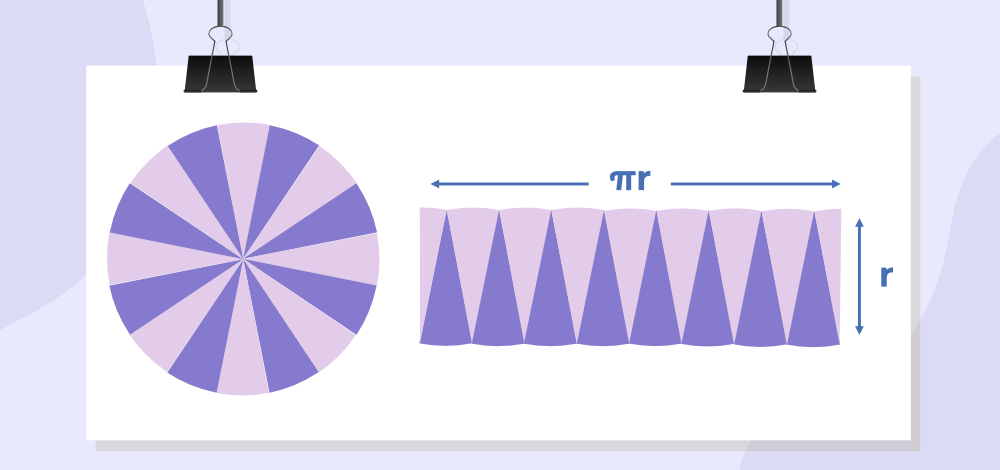After studying the above figure carefully, we split the circle into smaller parts and arranged them in such a way that they form a parallelogram. If the circle is divided into small and smaller parts, at last, it takes the shape of a rectangle.

Area of Rectangle = length × breadth

Comparing the length of a rectangle and the circumference of a circle we can see that the length is = ½ the circumference of a circle

Length of a rectangle = ½ × 2πr = πr

Area of circle = Area of rectangle = πr × r = πr2

Area of the circle = πr2

where
r is the radius of the circle.

### Circle’s Area Using Triangles

The area of the circle can easily be calculated by using the area of triangle. For finding the area of the circle using the area of the triangle consider the following experiment. Take a circle with a radius of r and fill the circle with concentric circles till no space is left inside the circle.

Now cut open each concentric circle and arrange them in a triangular shape such that the shortest length circle is placed at the top and the length is increased gradually.

The figure so obtained is a triangle with base 2πr and height r as shown in the figure given below,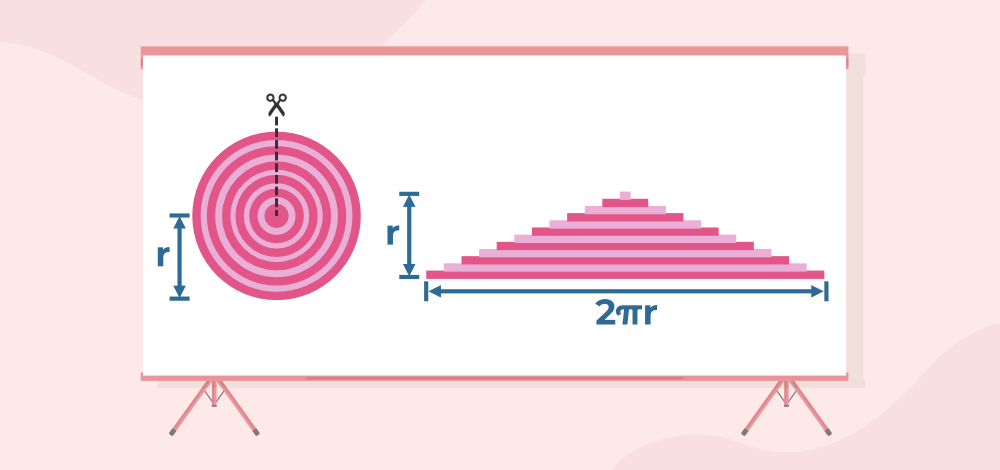Thus the area of the circle is given as,

A = 1/2 × base × height

A = 1/2 × (2πr) × r

A = πr2

## How to Find the Area of the Circle?

Various steps required to find the area of the circle are given below:

Step 1: Mark the radius of the circle.

Step 2: Put the value of the radius in the formula A = πr2, where r is the radius and π is the constant with a value of 3.14 (approx)

Step 3: Obtained the answer in step 2 is the required area of the circle. It is measured in square units.

If the diameter of a circle is given, it is first changed to radius using the relation Diameter = Radius / 2

## Area of a Sector of a Circle

Area of a sector of a circle is the space occupied inside a sector of a circle’s border. A semi-circle is likewise a sector of a circle, where a circle has two equal-sized sectors. Area of a sector of a circle formula is given below:

A = (θ/360°) × πr2

where,
θ is the sector angle subtended by the arcs at the center (in degrees),
r is the radius of the circle.

### Area of Quadrant of a circle

A quadrant of a circle is the fourth part of a circle. It is the sector of a circle with an angle of 90°. So its area is given by the above formula

A = (θ/360°) × πr2

Area of Quadrant = (90°/360°) × πr2
= πr2 / 4

## Differences Between Area and Circumference of a Circle

The basic difference between the area and the circumference of the circle is discussed in the table below,

## Real-World Examples on Area of Circle

We came across various examples which resemble circular shapes in our daily life. Some of the most common examples of the real-life circular things which we observe in our daily life are shown in the image below,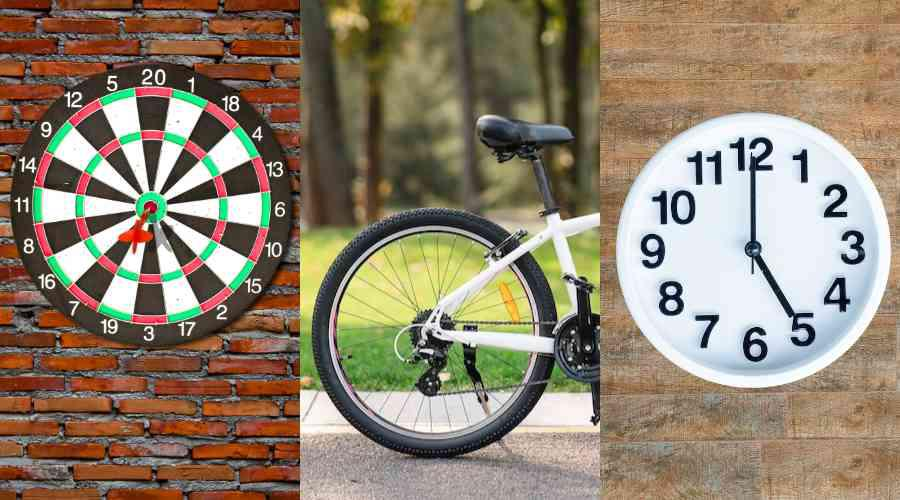## Solved Examples on Area of Circle

Example 1: A large rope is in a circular shape. Its radius is 5 units. What is its area?

Solution:

A large rope is in circular shape means it is similar to circle, so we can use circle formulae to calculate the area of the large rope.

given, r = 5 units, π = 3.14

Area = 3.14 × 5 × 5
=  78.50 unit2

Thus, the area of the circle is 78.50 units2

Example 2: If the rope is in a circular shape and its diameter is 4 units. Calculate its area.

Solution:

We know that rope is in circular shape, and its diameter = 4 units
π = 3.14

Area = (3.14 /4) × 4 × 4
= 12.56 units2

Therefore, the area of the rope is 12.56 units2

Example 3: If the circumference of the circle is 8 units. Calculate its area.

Solution:

Circumference of the circle = 8 units (given)

π = 3.14

Area = 8 × 8 / (4 × 3.14)
= 5.09 units2

Therefore, the area of the circle is 5.09 units2

Example 4: Find the circumference and the area of the circle if the radius is 21 cm.

Solution:

Circumferencer of the circle = 2πr cm.

Now, substituting the value, we get

C = 2  × (22/7)× 21
C = 2×22×3
C = 132 cm

Thus, circumference of the circle is 132 cm.

Now, area of the circle = πr2 cm2

A = (22/7) × 21 × 21
A = 22 × 63
A = 1386 cm2

Thus, area of the circle is 1386 cm2

Example 5: Find the area of the quadrant of a circle if its radius is 14 cm.

Solution:

Given r = 14 cm, π = 22 / 7

Area of quadrant =  πr2 / 4
= 22 / 7 × 142 × 1/4
= 154 cm2

Thus, the required area of quadrant = 154 cm2

Example 6: Find the area of the sector of a circle that subtends 60° angle at the center, and its radius is 14 cm.

Solution:

Given r = 14 cm, π = 22 / 7

Area of sector = (θ/360°) × πr2
= (60° /  360°) × 22 / 7 × 142
= 102.67 cm2

Thus, the required area of quadrant = 102.67 cm2

## FAQs on Area of Circle

### Q1: Write the formula for the circumference of a circle.

Circumference of circle is the boundary of the circle. Circumference can be calculated by multiplying the radius of circle with twice π. i.e. Circuference = 2πr.

### Q2: How to find the area of circle?

Area of a circle can be determined by using the formulas:

• Area = π x r2, where, r is radius of circle
• Area = (π/4) x d2,where, d is diameter of circle
• Area = C2/4π, where, C is circumference of circle

### Q3: What is meant by the area of the circle? Also, find the formula for it.

Area of circle is the space occupied by circle in the two-dimensional space. The formula for it is Area = π x r2

### Q4: What is Area of a Circle when the Diameter is given?

Diameter of the circle is twice the radius of the circle.The formula of the area of the circle, using the diameter of the circle is π/4 × diameter2.